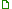A site run by veteran gamers with the aim of sharing discussions and content exclusively related to the development of Helbreath and its gameplay.Join Helbreath :: Server Development :: C++

# [FIX] Negative Stat PointsAuthorMessage
sleeqPosts : 22
Since : 2019-09-24Subject: [FIX] Negative Stat Points11/21/2019, 1:22 pm Repost from old helbreath.online forumThis bug appears when putting more than 200 points on each stat at one go. With this fix, you are able to put more than 10k points.HGServer SRCGame.cppFind in CGame::LevelUpSettingsHandler Code: char * cp, cStr, cVit, cDex, cInt, cMag, cChar;int iTotalSetting = 0;   Replace with Code: char * cp;// sleeq - fix for negative level up pointsshort * sp;short cStr, cVit, cDex, cInt, cMag, cChar;int iTotalSetting = 0;  Find Code: cStr = *cp;cp++;cVit = *cp;cp++;cDex = *cp;cp++;cInt = *cp;cp++;cMag = *cp;cp++;cChar = *cp;cp++; Replace with Code: sp  = (short *)cp;cStr  = *sp;cp += 2;sp  = (short *)cp;cVit  = *sp;cp += 2;sp  = (short *)cp;cDex  = *sp;cp += 2;sp  = (short *)cp;cInt  = *sp;cp += 2;sp  = (short *)cp;cMag  = *sp;cp += 2;sp  = (short *)cp;cChar  = *sp;cp += 2;     Client SRCGame.hFind Code: char m_cLU_Str, m_cLU_Vit, m_cLU_Dex, m_cLU_Int, m_cLU_Mag, m_cLU_Char;   Replace with Code: short m_cLU_Str, m_cLU_Vit, m_cLU_Dex, m_cLU_Int, m_cLU_Mag, m_cLU_Char; // sleeq - fix for negative level up points     Game.cppFind Code: case MSGID_LEVELUPSETTINGS:        // CLEROTH        //if ((m_cLU_Str + m_cLU_Vit + m_cLU_Dex + m_cLU_Int + m_cLU_Mag + m_cLU_Char) > 3) return FALSE;        dwp = (DWORD *)(cMsg + DEF_INDEX4_MSGID);        *dwp = dwMsgID;        wp  = (WORD *)(cMsg + DEF_INDEX2_MSGTYPE);        *wp = NULL;        cp = (char *)(cMsg + DEF_INDEX2_MSGTYPE + 2);        *cp = m_cLU_Str;        cp++;        *cp = m_cLU_Vit;        cp++;        *cp = m_cLU_Dex;        cp++;        *cp = m_cLU_Int;        cp++;        *cp = m_cLU_Mag;        cp++;        *cp = m_cLU_Char;        cp++;        iRet = m_pGSock->iSendMsg(cMsg, 12);        break;   Replace with Code: case MSGID_LEVELUPSETTINGS:        // sleeq - fix for negative level up points        dwp = (DWORD *)(cMsg + DEF_INDEX4_MSGID);        *dwp = dwMsgID;        wp  = (WORD *)(cMsg + DEF_INDEX2_MSGTYPE);        *wp = NULL;        cp = (char *)(cMsg + DEF_INDEX2_MSGTYPE + 2);        sp = (short *)cp;        *sp = (short)m_cLU_Str;        cp += 2;                sp = (short *)cp;        *sp = (short)m_cLU_Vit;        cp += 2;                sp = (short *)cp;        *sp = (short)m_cLU_Dex;        cp += 2;                sp = (short *)cp;        *sp = (short)m_cLU_Int;        cp += 2;                sp = (short *)cp;        *sp = (short)m_cLU_Mag;        cp += 2;                sp = (short *)cp;        *sp = (short)m_cLU_Char;        cp += 2;        iRet = m_pGSock->iSendMsg(cMsg, 6 + 12);        break;[FIX] Negative Stat PointsPage 1 of 1

Permissions in this forum:You cannot reply to topics in this forumJump to: Select a forum||--Popular|   |--General|   |--Ask the Community|   |--Server Development|   |--Guides|   |--Files / Sources|   |--C++|   |--Sprites|   |--Tools / PHP|   |--Visuals & Videos|   |--Miscellaneous    |--Canceled Posts